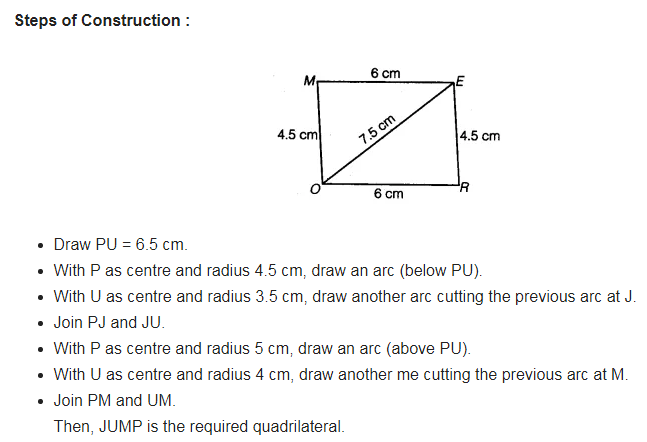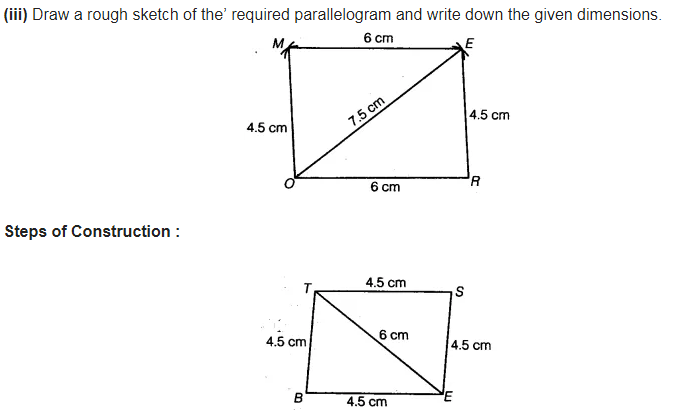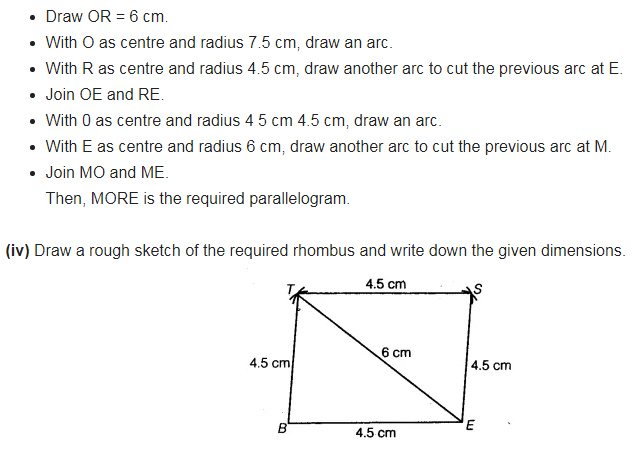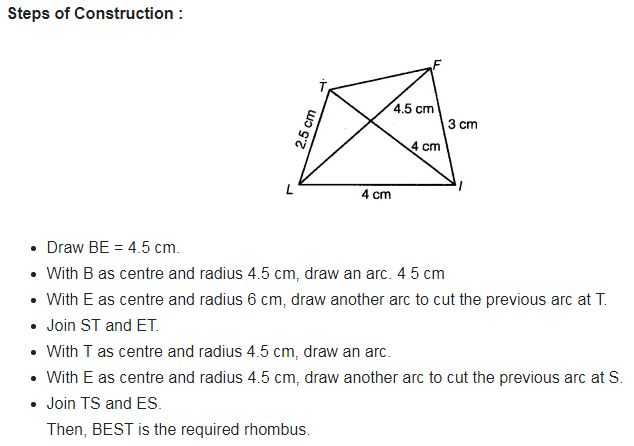AB = 4.5 cm
BC = 5.5 cm
CD = 4 cm
AC = 7 cm

JU = 3.5 cm
UM = 4 cm
MP = 5 cm
PJ = 4.5 cm
PU 6.5 cm

(iii) Parallelogram MORE
OR = 6 cm
RE = 4.5 cm
EO = 7.5 cm

(iv) Rhombus BEST
BE = 4.5 cm
ET = 6 cm